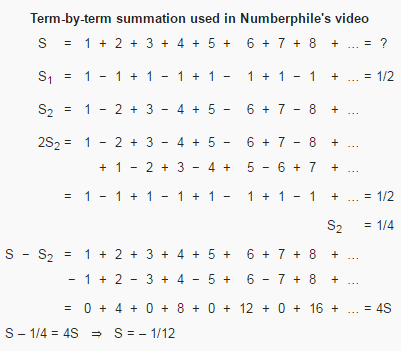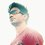# Interesting misconception - which one is true?$\displaystyle \zeta(n)=\sum_{k \geq 1} k^{-n}$

We know that $\zeta(-1)=\frac{-1}{12} , \zeta(2)=\frac{\pi^2}{6}$

The point is that

$\displaystyle \sum_{n\geq 1}n+\frac{1}{n^2}$ does not converge according to wolframalpha.

But by using the definition of the Riemann zeta function,

$\displaystyle \sum_{n\geq 1}n+\frac{1}{n^2}=\frac{-1}{12}+\frac{\pi^2}{6}$

Which is the true statement?Note by Aman Rajput
4 years, 5 months ago

This discussion board is a place to discuss our Daily Challenges and the math and science related to those challenges. Explanations are more than just a solution — they should explain the steps and thinking strategies that you used to obtain the solution. Comments should further the discussion of math and science.

When posting on Brilliant:

• Use the emojis to react to an explanation, whether you're congratulating a job well done , or just really confused .
• Ask specific questions about the challenge or the steps in somebody's explanation. Well-posed questions can add a lot to the discussion, but posting "I don't understand!" doesn't help anyone.
• Try to contribute something new to the discussion, whether it is an extension, generalization or other idea related to the challenge.

MarkdownAppears as
*italics* or _italics_ italics
**bold** or __bold__ bold
- bulleted- list
• bulleted
• list
1. numbered2. list
1. numbered
2. list
Note: you must add a full line of space before and after lists for them to show up correctly
paragraph 1paragraph 2

paragraph 1

paragraph 2

[example link](https://brilliant.org)example link
> This is a quote
This is a quote
    # I indented these lines
# 4 spaces, and now they show
# up as a code block.

print "hello world"
# I indented these lines
# 4 spaces, and now they show
# up as a code block.

print "hello world"
MathAppears as
Remember to wrap math in $$ ... $$ or $ ... $ to ensure proper formatting.
2 \times 3 $2 \times 3$
2^{34} $2^{34}$
a_{i-1} $a_{i-1}$
\frac{2}{3} $\frac{2}{3}$
\sqrt{2} $\sqrt{2}$
\sum_{i=1}^3 $\sum_{i=1}^3$
\sin \theta $\sin \theta$
\boxed{123} $\boxed{123}$

Sort by:

The problem with the Numberphile video is that you can deduce all sorts of things if you mis-manipulate $\infty$. Such as $\begin{array}{rcl} \infty + 1 & = & \infty \\ 1 \; = \; (\infty + 1) - \infty & = & \infty - \infty \; = \;0 \end{array}$ The Numberphile video introduces $s$, which is equal to $\infty$. It then says that $s_1 = \tfrac12$. Neither of these series converge. The first diverges, and the partial sums of the second alternate between $0$ and $1$. This does not make the average of these values equal to the limit. If you start with $\infty$, and a false statement, you can deduce anything.

These manipulations are formal, and are not correct in themselves. You can (formally) get $s = -\tfrac{1}{12}$ by putting $s=-1$ into $\zeta(s)$, and you can (formally) get $s_1 = \tfrac12$ by putting $z=-1$ into $(1-z)^{-1}$. Both of these "results" are consequences of two different ways of expressing functions obtained by analytic continuation, equating the true value with the incorrect application of the formula defining the function on some subdomain.

- 4 years, 5 months ago

okay... thanks sir :)

- 4 years, 5 months ago

Complex function theory introduces the concept of analytic continuation. It is possible to define a function on a subset of the complex plane, and then extend to it analytically to a larger subset of the plane. That does not mean that the original formula used to define the function is valid on the larger domain.

The function $(1-z)^{-1}$ is analytic on the whole complex plane except for $1$, but is only defined by the power series expansion $(1 - z)^{-1} \; = \; \sum_{n=0}^\infty z^n$ when $|z| < 1$. You would not try to apply the series expansion for other values of $z$, and say $-1 \; = \; \sum_{n=0}^\infty 2^n$ for example.

Or consider the Gamma function. It is defined for all complex $z$ with strictly positive real part by the integral formula $\Gamma(z) \; = \; \int_0^\infty t^{z-1} e^{-t}\,dt \qquad \qquad \mathrm{Re}\,z > 0$ For all $z$ with $\mathrm{Re}\,z > 0$, we can prove, using this integral definition, that $\Gamma(z+1) \; = \; z \Gamma(z) \qquad \qquad \mathrm{Re}\,z > 0$ So far, so good.

We can use this formula to extend the Gamma function to all complex numbers with real part greater than $-1$, except $0$, by the formula $\Gamma_1(z) \; = \; \frac{\Gamma(z+1)}{z} \qquad \qquad \mathrm{Re}\,z > -1 \;, \qquad z \neq 0$ It is clear that $\Gamma_1$ is analytic on this new domain, and coincides with $\Gamma$ on the domain of $\Gamma$. Moreover, we can show that $\Gamma_1$ satisfies the equation $\Gamma_1(z+1) \; =\; z\Gamma_1(z) \qquad \qquad \mathrm{Re}\,z > -1 \;, \quad z \neq 0$

We can now extend $\Gamma_1$ to a new analytic function $\Gamma_2$ on the set of all complex numbers with real part greater than $-2$ except $-1,0$ by the formula $\Gamma_2(z) \; =\; \frac{\Gamma_1(z+1)}{z} \qquad \qquad \mathrm{Re}\,z > -2 \;, \qquad z \neq -1,0 \;.$ We can keep on going, and obtain a sequence of analytic functions $\Gamma_n$, where each $\Gamma_{n+1}$ is an extension of $\Gamma_n$, on increasingly large domains.

It is then possible to put these all together uniquely as an analytic function with domain the whole of $\mathbb{C}$ except for $0,-1,-2,-3,\ldots$, which extends the original Gamma function. This final extension is what we call the Gamma function. To save effort, we denote all these functions by the single symbol $\Gamma$.

However, there is no reason to suppose that the integral formula for $\Gamma(z)$ works except when $z$ has positive real part - it is not valid anywhere else.

The Riemann zeta function is defined by the formula $\zeta(s) \; = \; \sum_{n=1}^\infty \frac{1}{n^s} \qquad \qquad \mathrm{Re}\,s > 1$ It can be extended analytically to the whole complex plane. That does not mean that the above infinite sum formula is valid elsewhere in the complex plane. In particular, while $\zeta(-1)=-\tfrac{1}{12}$, we cannot use this to sum the positive integers to infinity.

The problem is that theoretical physicists seem to do so! They use formulae like $1 + 2 + 3 + \ldots = -\tfrac1{12}$ in high-powered quantum renormalization arguments, and get the right answer. They do so because what they are doing is a short-hand for using the zeta function properly, moving from the infinite sum (for values of $s$ where it is valid), recognising that this is the zeta function, and that their theory is valid for all complex numbers $s$, and then allowing themselves to put $s=-1$, using the correct formula $-\tfrac1{12}$ for $\zeta(-1)$. Just writing $1 + 2 + 3 + \cdots = -\tfrac{1}{12}$ saves a couple of pages of algebra/analysis! That does not make the formula true

- 4 years, 5 months ago

i know that this relation is widely useful in quantum and string theories. But i am not able to understand the validity of these two .. where to use this equation or where not ???

- 4 years, 5 months ago

You can only use this formula if you can take the series $\sum n$, replace it by $\zeta(s)$, do the analysis for general $s$, and then put $s=-1$ in the final result.

A lot of quantum and string theory calculations involve tricks such as these, which are often first introduced "by intuition", or because they are necessary to make things work. A more careful later analysis does the work accurately. Basically, what is happening is that the starting model of the physics of a problem leads to a situation where we are asked to sum the positive integers. A more carefully-formed model would ask us to evaluate $\zeta(s)$. A simplified approach would be simply to work with summing the integers, and using the answer $-\tfrac{1}{12}$. However, to do so is just to adopt a notational shorthand.

Physicists are quite fond of simplified models, even though they are nonphysical. For example, if you study the energy levels of the hydrogen atom, you will work with a $1/r$ potential, even though that is not physical (it is not valid as $r \to 0$, since it does not account for the dimensions of the proton). However, it is a model which has exact solutions, and is a good first approximation to the true situation. It is a weirdness of quantum field theory that fixing some of these models requires us to appear to play fast and loose with infinity.

- 4 years, 5 months ago

okay thank you sir

- 4 years, 5 months ago

The value of $\zeta (-1)$ is found via analytic continuation. In fact, the following equation : $\zeta (s) = \sum_{n=1}^{\infty} \frac{1}{n^s}$ is valid only if $Re(s)>1$.

For the value at other points, we need to use analytic continuation.

- 4 years, 5 months ago

one can also find the $\zeta(-1)=-1/12$ using the standard definition. i dont think so $Re(s)>1$ . I can prove that...

- 4 years, 5 months ago

If by standard definition you mean the series, then it's wrong as $\lim_{n \to \infty} \sum_{r=1}^{n} r = \lim_{n \to \infty} \frac{n(n+1)}{2} \to \infty$

- 4 years, 5 months ago

let me show you :) what i want to say .. check the note again . i used the standard definition

- 4 years, 5 months ago

In fact with some more manipulations, you can show that $0=1$ as well.

- 4 years, 5 months ago

oh nice... that i am surely like to see. but show me using the above image :)

- 4 years, 5 months ago

$1+2+3 \cdots = -\frac{1}{12} \\ 0+1+2 \cdots = -\frac{1}{12} \\\implies 1+1+1 \cdots = -\frac{1}{12} - \left( -\frac{1}{12} \right)=0\\ 0+1+1 \cdots = 0 \\ by \, subtracting, \, 1=0$

- 4 years, 5 months ago

but 1+1+1+1... = -1/2

- 4 years, 5 months ago

But $1=0$. Hence, $LHS=0$ and $RHS=-\frac{0}{2}=0$ ;)

- 4 years, 5 months ago

hahahaha.. nice

- 4 years, 5 months ago

Also, to continue the joke, $1+2+3 \cdots = -\frac{1}{12} = -\frac{0}{12} =0$

- 4 years, 5 months ago

$s_1$ doesn't exist. That's the first flaw in all such proofs. ;)

- 4 years, 5 months ago

i can prove... s1 also

- 4 years, 5 months ago

First prove it exists. Then we'll go about calculating it.

- 4 years, 5 months ago

- 4 years, 5 months ago

"We know that $\zeta(-1) = -\dfrac{1}{12}$" That's a regularized value of the series. The series doesn't converge.

- 4 years, 5 months ago

what do you mean by regularised value :D

- 4 years, 5 months ago